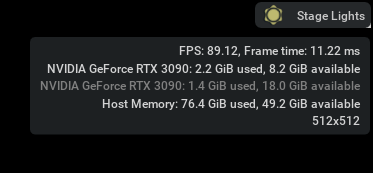# IMU topic publish Code error

I wrote the python code to publish the imu topic in Isaac Simulator like below code. And I set the sensor_period to 1/400, which is the 400 hz. But I got the UI FPS(like below image 89), not 400.

I run below code using script editor in Isaac Simulator.
What’s the matter in my code? And why am I getting from UI FPS, not sensor_period?

My Isaac version is “2022.2.0” and ros version is Noetic.
Thank you so much for reading this problem!

``````import rospy
import asyncio
from omni.isaac.sensor import _sensor
import omni
from sensor_msgs.msg import Imu
import omni.kit.commands
import time
from pxr import Gf, UsdGeom

class ImuPub:
def __init__(self):

self.imu_msg = Imu()
isaac_imu_topic = "/carter1/imu"
self.isaac_imu_pub = rospy.Publisher(isaac_imu_topic, Imu, queue_size=10)
self._is = _sensor.acquire_imu_sensor_interface()
result, sensor = omni.kit.commands.execute(
"IsaacSensorCreateImuSensor",
path =  '/Carter_imu',
parent = self.carter_usd_path,
sensor_period = 1/400,
translation = Gf.Vec3d(0,0,0),
orientation = Gf.Quatd(1, 0,0,0),
visualize = False, )

self.meters_per_unit = 1.0

print("Initialization Complete")

def run(self):
rospy.init_node("isaac_imu_pub")
asyncio.ensure_future(self.pub_imu())

async def pub_imu(self):
process_min = 0.5
t_end = time.time() + (60 * process_min)

while time.time() < t_end:
#		while not rospy.is_shutdown():
await asyncio.sleep(0.0001)
isaac_imu_msg = Imu()

self.isaac_imu_pub.publish(isaac_imu_msg)

if __name__ == "__main__":
imupub = ImuPub()
imupub.run()
``````Hi @phr0201 - Someone from our team will review the question and respond back to you.

hi, I have this question, too. Did you solved it?

Hello,

thanks for reaching out to the forum. I think the issue is that the IMU is called by the render callback (by default), not the physics callback. To do so, you need to first instantiate the world with physics rate based on your desired IMU frequency (1/400). For example, the snippet below will create a world with physics rate being 400hz, rendering at 50fps

`my_world = World(stage_units_in_meters=1.0, physics_dt=1 / 400, rendering_dt=1 / 50)`

then create a physics call back in your constructor to get the IMU data and publish it each physics step
`my_world.add_physics_callback("sensor_measurement", onPhysicsStep)`

``````def onPhysicsStep(step_size):
self.pub_imu()
``````

Let us know if this workes Thanks!